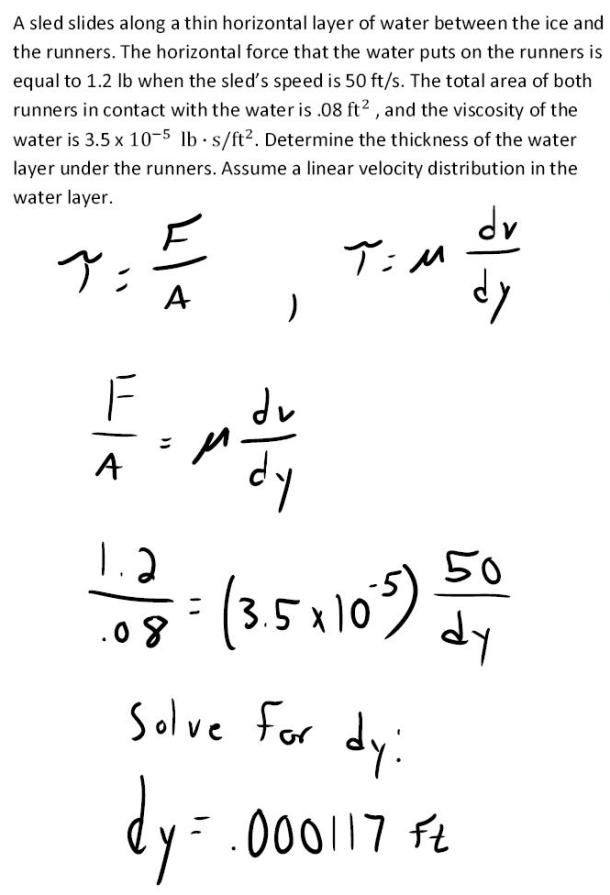fluid mechanics math problems engineering equations fluid mechanics basic mechanics school homework engineering math fluids formulas fluid mechanics problems fluid mechanics problem solutions to fluids problems full solution fluid mechanics engineering fluids problem solution fluid mechanics math problems engineering equations fluid mechanics basic mechanics school homework engineering math fluid mechanics formulas fluid problems fluid mechanics problem solutions to fluid mechanics problems full solution fluid mechanics engineering fluid mechanics problem solution
fluid mechanics math problems engineering equations fluid mechanics basic mechanics school homework engineering math fluids formulas fluid mechanics problems fluid mechanics problem solutions to fluids problems full solution fluid mechanics engineering fluids problem solution fluid mechanics math problems engineering equations fluid mechanics basic mechanics school homework engineering math fluid mechanics formulas fluid problems fluid mechanics problem solutions to fluid mechanics problems full solution fluid mechanics engineering fluid mechanics problem solution
Highalphabet Home Page fluid mechanics solutions fluids math solved Fluid Mechanics Page 1
A sled slides along a thin horizontal layer of water between the ice and the runners. The horizontal force that the water puts on the runners is equal to 1.2 lb when the sled's speed is 50 ft/s. The total area of both runners in contact with the water is .08 ft^2, and the viscosity of the water is 3.5x10^-5 lb s/ft^2. Determine the thickness of the water layer under the runners. Assume a linear velocity distribution in the water layer.A sled slides along a thin horizontal layer of water between the ice and the runners. The horizontal force that the water puts on the runners is equal to 1.2 lb when the sled's speed is 50 ft/s. The total area of both runners in contact with the water is .08 ft^2, and the viscosity of the water is 3.5x10^-5 lb s/ft^2. Determine the thickness of the water layer under the runners. Assume a linear velocity distribution in the water layer.